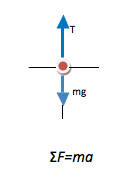# University of Wisconsin Green Bay

### Dynamics (Force or Newton’s 2nd Law) Problems

Dynamics (Force) problems ask you to relate motion to the forces causing it. Note that the word “force” isn’t always used explicitly in the statement of the problem. You know many forces such as gravity, tension, and normal force that are present even if not listed in the problem.

#### How to Solve Force Problems

• ##### 1.Identify the ProblemAny problem that asks you to relate force and motion is a Newton’s Second Law problem, no matter what was given or requested in the problem. In some cases, Newton’s Second Law is easy to identify—for example, a problem might ask you for the value of a particular force.

In other cases, it is harder to recognize 2nd Law problems. You may know forces that are present without having force ever mentioned in the problem, and you may be asked for things like how fast an object moves in a circle or how much of an object is underwater. Recognizing how to approach these problems comes from experience—taking the time to answer the question “how did I know this was a 2nd Law problem” for every single force example you work will help to build that intuition which you will need on the final exam.

• ##### 2. Draw a Picture

When you approach force problems, a free body diagram will allow you to both picture what is happening and directly map the picture into the equation. All the physics is done in the process of drawing the diagram—only algebra remains after this step.

The first thing that you will need to do is to identify what object(s) will be the focus of the diagram—in other words, what system do you need to consider in order to answer the question. In some cases, you will need to consider several objects as separate systems. In those cases, you will draw free body diagrams and set up equations for each object separately. You will very likely use Newton’s Third Law to relate forces on the two objects.

Once you have identified your system, think about all forces on that system, discard any that are too small to matter. Only forces acting on the object should be shown, since you are trying to understand what causes the motion of the object. The free body diagram maps directly into the left side of ∑F=ma. Acceleration is the result not the cause—if you wish to sketch the acceleration, make sure that you do so off to the side and not on the sketch of forces.

Because Newton’s Second Law is a vector equation, you will need to divide all forces into their x- and y- components in order to work with the equation. Math is always easiest if you pick one axis to be along the direction of acceleration. That way, one component of a will be zero and you will have fewer linked equations.

• ##### 3. Select the RelationAll force problems begin with the relation ∑F=ma. This true no matter what you are asked to find. If any additional information is needed, it will become apparent as you work through the problem.

• ##### 4. Solve the Problem

∑F=ma is a vector equation and so is set up separately in the x- and y- directions. Think carefully about the direction of each force and include the appropriate sign. Once you have filled your forces into these equations, you have only algebra left and can solve the equations in any way that works. In general, it is easiest to solve the equation in which a=0 first as you will sometimes need those values in the other expression.

• ##### 5. Understand the Results

Once you have completed the problem, look at it again. Does your answer make sense? Did it give the behavior you intuitively expected to find? Can you now do steps that caused you problems earlier? Can you explain in words what is happening? If you only recognized it as a 2nd Law problem because of the section heading in your text book, identify the information that you would use to recognize a problem like this on the final exam.

#### Help! I can’t find an example that looks like the problem I need to work!

• ##### Are you certain your problem is a Newton’s Second Law problem?

One of the most common mistakes is to think too hard. If you are told net force and asked for acceleration, or vice versa, you don’t need to go through all of the steps of a Newton’s Second Law problem. Check Definition and Ratio problems to see if you can find a useful example.

It is also possible that your problem is better solved using kinematics (description of motion) or energy and momentum. Can you clearly explain to yourself that your problem requires you to relate the cause of motion (forces) to the effect of those forces (acceleration or changing velocity?)